• 0

# Probability Plot

Probability Plot is a graphical tool for to assess if the data follows a particular distribution or not. Data is plotted against a hypothesized distribution and if it roughly forms a straight line, it indicates that data follows the hypothesized distribution. Deviation from straight line indicates that data does not follow the hypothesized distribution.

An application-oriented question on the topic along with responses can be seen below. The best answer was provided by Vidhya Rathinavelu on 23rd May 2023.

Applause for all the respondents - Vidhya Rathinavelu, Gitarchana Roy, Moushmi Kandori, Khalandar S, Raghavendra Rao Althar, Ajay Sharma, Sanjay Bhure, Nikita Chordia.

## Question

Q 567. Explain how a probability plot helps us assess the distribution of the data. What insights do we get from a curve that deviates from a straight line in a probability plot?

Note for website visitors -

## Recommended Posts

• 1

A probability plot is the graphical method for validating the distribution of data. It is a line plot of the data points against the theoretical values, which are the values of a cumulative distribution function, of a probability distribution.

A probability plot can be used to assess the shape, location, Spread & outliers. It can also be used to compare the distributions of two or more sets of data.

To create a probability plot, the data points are first arranged in ascending order. Then the cumulative distribution function is calculated and data is plotted.

A well-fitting probability plot will have a straight line. If the data points are scattered around the line, then the distribution is not well-represented

Some of the insights that we can get from a curve that deviates from a straight line are that, data:

• is not normally distributed.
• has outliers.
• may be skewed.
• Does not facilitate use of standard statistical methods to analyze the data.
• requires transformation or use non-parametric statistical methods.

Regards

Vidhya R

##### Share on other sites

• 0

A probability plot is a graphical technique for assessing whether the data set follows a normal distribution or not. The normal probability plot is also a type of Quantile plot. It is a graph used to assess the Process Capability i.e., if a straight line is plotted we say the process is normally distributed.

The probability plot is always plotted by arranging data in the ascending order and creating quantiles.

Insights from the graph

Deviation from this straight line denotes deviation from normality. This usually happens if there are outliers in the data set.

Skewness in the data set denotes asymmetrical data. Positive skewness denotes longer right tail and negative skewness denotes longer left tail.

Kurtosis describes how much the probability distribution falls in the tails instead of its center.

There can be 2 insights for kurtosis i.e., heavy tailed or Light tailed distribution.

·      If the curve bends upward more than expected in the upper quantiles or bend downward more than expected in the lower quantiles then it is a heavy tailed distribution or it has a higher probability of extreme values as compared to light tailed distributions.

·      If the curve bends downward more than expected in the upper quantiles or bends upward more than expected in the lower quantiles then it is a light tailed distribution i.e., lower probability of extreme values as compared to heavy tailed distributions.

##### Share on other sites

• 0

A probability plot is a visual tool for analysing the distribution of data. The cumulative distribution function (CDF) of the data is plotted against a reference line on a line graph. The CDF is a step function that leaps from 0 to 1 at each data point, and the reference line is often a straight line.

The probability plot's form can be used to evaluate the data's distribution. For instance, if the data are regularly distributed, the probability plot will be a straight line. The probability plot will be curved if the data is skewed.

Outliers can also be discovered using probability graphs. Outliers are data points that deviate significantly from the average. Look for data points that are significantly above or below the reference line to spot them.

A helpful tool for analysing the distribution of data is a probability plot. They can be used to determine the distribution's shape, spot outliers, and assess how two or more data sets' distributions compare.

A probability plot is a visual tool for evaluating how well a collection of data fits a distributional model. A probability map with a straight line shows that the hypothesised distribution fits the data well. A curve that deviates from a straight line shows that the hypothesised distribution does not adequately fit the data.

On a probability plot, a curve that deviates from a straight line may occur for a variety of reasons. Among them are the following:

·         The proposed distribution does not adequately capture the observed data.

·         Outliers contaminate the data.

·         The distribution that was predicted is unreliable.

It is crucial to look into the causes of any deviations from a straight line that appear on a probability plot. To achieve this, examine the data, search for outliers, and experiment with various hypothesised distributions.

##### Share on other sites

• 0

Normal Probability Plots are used to check whether or not a data set follows hypothesized distribution.

Normal Probability plot has two versions : Q-Q & P-P.

Q-Q Plot: Q - Quantile means that dividing the data set into Equal parts or Equal subgroups. The reason behind calling it as Q-Q Plot is that it takes the observed data on one axis & values derived from standard normal distribution with same data points on other axis. If the data  is normally distributed, the probability plot will be a straight line. If the data is skewed, the probability plot will be curved.

The Q-Q Plot plots the Quantiles of Actual data set against to theoretical values of Data set under normal distribution. These plots are used to find out the deviations in the tails of data distribution.

P-P Plot: Probability - Probability Plot plots the cumulative distribution functions of data set (Empirical on one axis vs specified theoretical on other axis). These plots are widely used to find out the deviations from normality in Centre of data distribution.

Below image is one of the good explanation to understand the Probability Curve vs Data distribution of various data sets.

There are various reason why a curve has deviation from a straight line on a Probability. Some of the reasons may be:

1. The data set is not well represented & distributed
2. The data set contain outliers (good & bad)
3. The data distribution is incorrect

Its important and recommended that if a curve deviated from Straight line on a Probability plot, we should investigate the root cause for the deviations through data examination, analysis on Outlier(s) & trying different hypothesized distributions.

##### Share on other sites

• 0

Probability plot helps to assess normality of the data. Outliers, skewness, kurtosis characteristics of the data can be understood. Kurtosis represents the characteristics of the tail part of the distribution. In case of the data distribution is close to the straight line representation in probability plot, data is normal. In probability plot theoretical quantiles are chosen to provide an approximation of mean or median of data distribution. Quantiles are range of probability distribution that are divided into continuous intervals with equal probability. Data points that are on upper or lower extreme of the line represents outliers of the data. These outliers influence the prediction modeling capabilities for the data set. Probability plots done on residual values of the data is helpful for purpose of prediction model validation and figure out outliers that cause test of models to fail. Probability plots also assist in DOE (Design Of Experiment) by effect plots that represents interaction between various factors in the experiment. Effects that are closer to normal probability line are not significant as they are part of random variations. We need to look at outliers that has significant influence.

##### Share on other sites

• 0

A probability plot, also known as a quantile-quantile (Q-Q) plot, is a graphical tool used to assess the distribution of data. It compares the observed data quantiles to the expected quantiles of a specified theoretical distribution, typically assuming a normal distribution.

To construct a probability plot, we sort the data in ascending order and calculate the corresponding quantiles. These quantiles represent the probabilities below which a certain percentage of the data falls. For example, the 25th percentile corresponds to the value below which 25% of the data lies.

Next, we determine the expected quantiles based on the theoretical distribution we want to compare against. For instance, if we assume a normal distribution, we calculate the expected quantiles using the inverse of the cumulative distribution function (CDF) of the normal distribution.

Plotting the observed quantiles against the expected quantiles produces a scatter plot. If the data closely follows the assumed distribution, the plot will exhibit a roughly straight line. Deviations from a straight line can provide valuable insights into the distribution of the data.

Here are a few insights we can gain from a curve that deviates from a straight line in a probability plot:

1. Skewness: If the curve deviates from a straight line in the tails, it suggests that the data might have a skewed distribution. Positive skewness occurs when the tail on the right side of the distribution is longer or fatter than the left side, while negative skewness is the opposite.

2. Heavy-tailed distribution: If the curve deviates upward in the middle or near the ends, it indicates heavy tails in the distribution. Heavy-tailed distributions have more extreme values than expected in comparison to the assumed distribution.

3. Light-tailed distribution: Conversely, if the curve deviates downward in the middle or near the ends, it suggests a light-tailed distribution. Light-tailed distributions have fewer extreme values than expected.

4. Deviation in the center: If the curve deviates from a straight line in the central portion, it might suggest a different location or spread than what is expected under the assumed distribution. This can indicate issues such as outliers, a shift in the mean, or a difference in variability.

By examining the deviations from the straight line in a probability plot, we can gain insights into the shape, skewness, tail behavior, and location of the data distribution. This information helps us assess the appropriateness of the assumed distribution and guides further analysis and modeling decisions.

##### Share on other sites

• 0

Probability Plot is a graphical method for determining weather sample data confirm to a given distribution based on a examination of the data. This given distribution is hypothesized distribution and the examination is subjective visual examination.
The observations in the sample are first ranked in ascending order (i.e. smallest to largest). The plotted points shall fall approximately along the straight line. The determination of weather or not the data plot as a straight line is subjective.

The graph is plotted between the observed data (in X axis) and cumulative % of probability (in Y axis). The probability is calculated using formula = (j-0.5)/n, i is the serial number of the observation and n is the total number of observations.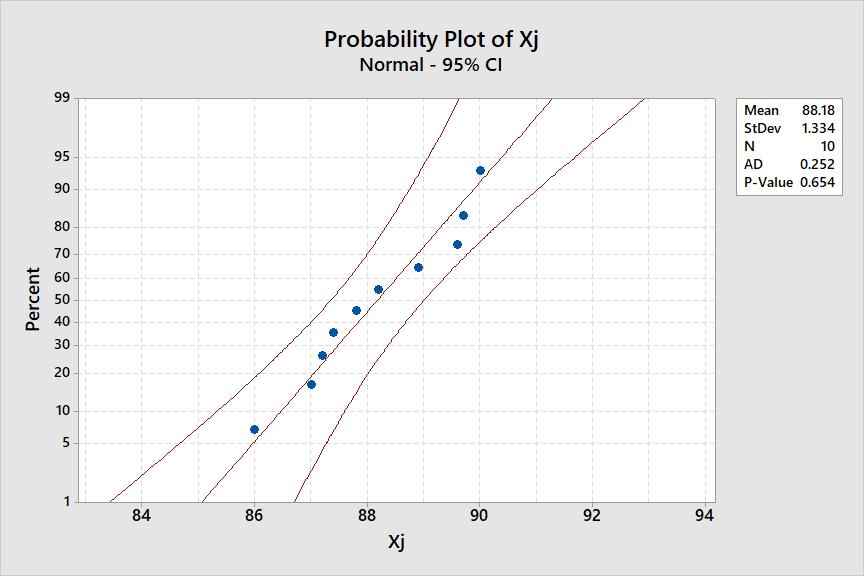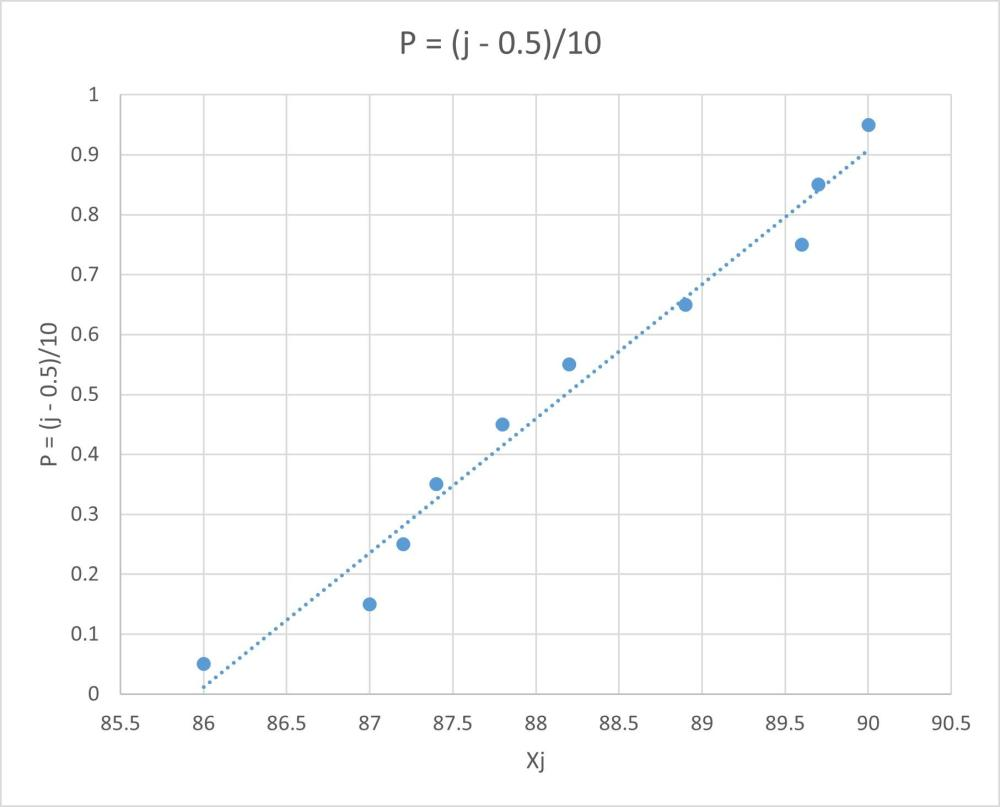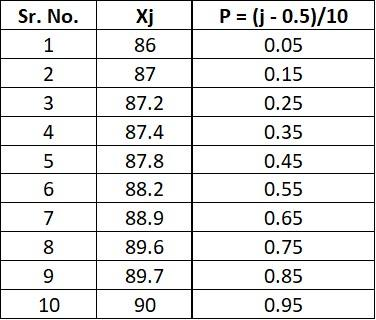##### Share on other sites

• 0

Probability plot

A probability plot is a simple tool to visually compare data coming from different datasets and determine whether or not a data set follows a hypothesized distribution.

Types of probability plots:

1.       P-P plot

The P-P (probability–probability) plot is a visualization that plots CDFs (cumulative distribution function) of the two distributions (empirical and theoretical) against each other.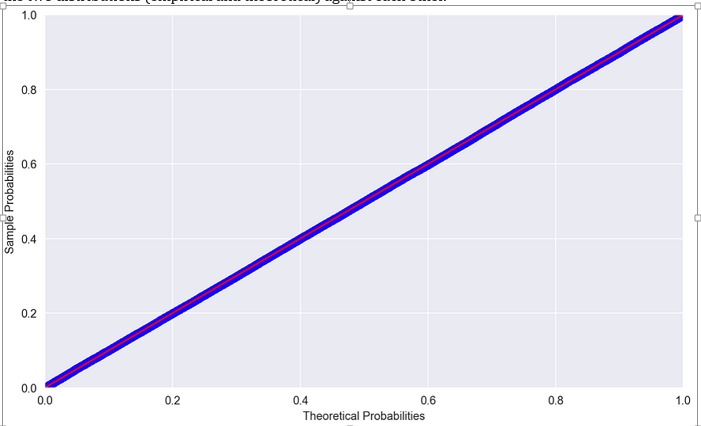Figure 1: Example of a P-P plot comparing random numbers drawn from N(0, 1) to Standard Normal — perfect match

1.       Q-Q plot

The q-q (Quantile-to-Quantile) plot is used to compare the quantiles of two distributions (empirical and theoretical) against each other.

Assessing the distribution of data:

·         If the data plotted against the theoretical distribution, results in a straight line, then the hypothesized distribution may be correct.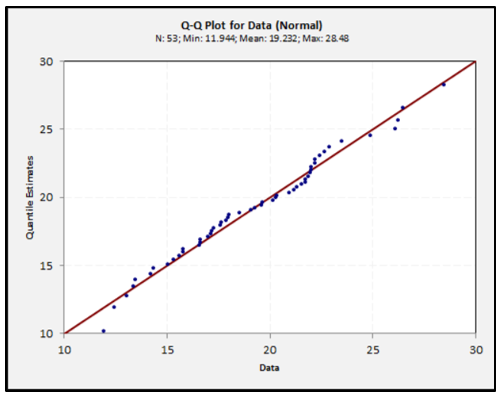·         If the data results in a curve, then it indicates that an asymmetrical distribution (non-normal) would be more appropriate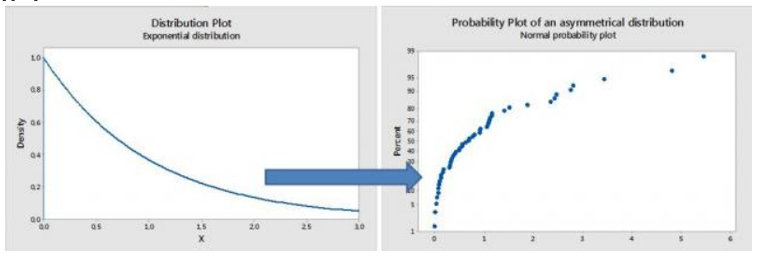For such non-normal distribution, we need to transform the data into normal distribution or identify appropriate distribution before performing analysis like capability study, regression etc.

·         If the plot represents distinct groups of data, then the sample set is discrete data.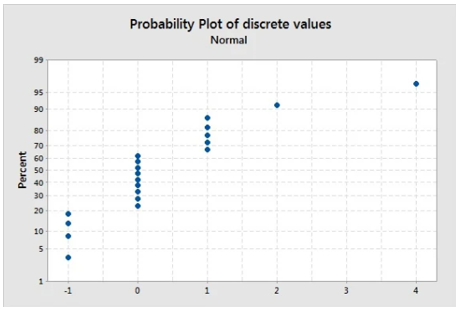##### Share on other sites

• 0

Very informative answers. It was a tought one to decide. The best answer has been given by Vidhya Rathinavelu. Answers from Gitarchana, Khalandar and Ajay are also a must read.

## Create an account

Register a new account

• ### Forum Statistics

• Total Topics
3.2k
• Total Posts
16.1k
• ### Member Statistics

• Total Members
54,703
• Most Online
990

×
×
• Create New...